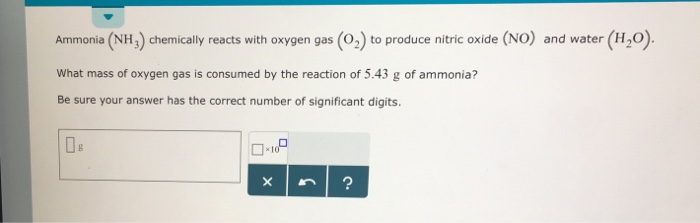# Question & Answer: Ammonia (NH_3) chemically reacts with oxygen gas (O_2) to produce nitric oxide (NO) and…..Ammonia (NH_3) chemically reacts with oxygen gas (O_2) to produce nitric oxide (NO) and water (H_2O). What mass of oxygen gas is consumed by the reaction of 5.43 g of ammonia? Be sure your answer has the correct number of significant digits.

WHAT mass of oxygen gas is consumed by the reaction of 5.43 g of ammonia

Balanced equation:

Don't use plagiarized sources. Get Your Custom Essay on
Question & Answer: Ammonia (NH_3) chemically reacts with oxygen gas (O_2) to produce nitric oxide (NO) and…..
GET AN ESSAY WRITTEN FOR YOU FROM AS LOW AS \$13/PAGE

4 NH3 + 5 O2 → 4 NO + 6 H2O

molar masses: O2= 32 g/mol

NH3= 17g/mol

Molar mass of 4NH3 = 68.1221 g/mol

molecular weight of 5O2=159.994

4NH3 —————–5O2

5.43 g of ammonia=0.3188393542886509 moles ammonia

Or

Number of moles =5.43/17=0.319=0.32 moles of ammonia

4NH3 —–consumes —————————5O2

0.32 moles of ammonia consumes ————??

Number moles of oxygen = (0.32 /4)*5=0.4 moles of oxygen

0.4 moles of oxygen =0.4*32= =12.8 grams answer

(5.43 /68.1221)*160=12.7535 grams